# What is Pi?

In this fun lesson, we will learn about what is pi (pronouced as pie), the pi symbol, the pi number, and the pi circle in the concept of what is pi. Check-out the interactive simulations to know more about the lesson and try your hand at solving a few interesting practice questions at the end of the page. So, what are you waiting for? Let's have a slice of this pi.

Did you know that the Ancient Greeks attempted to study pie in 2000 BC? Over millennia, these are the inferences that have been derived that include the fact that pi is an irrational number, it is teh ratio of the circumference of the circle to its diameter.

The pi symbol is $$\pi.$$ It is also called Archimedes' constant after the Greek mathematician, Archimedes, who created an algorithm to approximate the pi value. The simulation below shows the circumference of a circle whose diameter is $$1 \: \text{cm.}$$

## Lesson Plan

 1 What is Pi? 2 Important Notes on Pi 3 Solved Examples on Pi 4 Tips and Tricks 5 Interactive Questions on What is Pi

## What is Pi in Maths?

We all know that pi value is constant. It is an irrational number usually approximated to $$3.14$$.

Let us now do a small activity to know what is pi exactly.

Draw a circle of any radius.

Use a thread and measure the diameter.

Now, bend this thread to form a curve and place it along the circumference of the circle.

You can also see this in the simulation below. Click on start animation to know how to do the activity.

## Formula for Pi

Now that we have understood what is pi, and we have observed that the pi value is constant, let us see how to calculate it.

Pi is the ratio of the circumference of a circle to its diameter. The formula for calculating $$\pi$$ is:

 \begin{align} \pi = \frac{\text{Circumference}}{\text{Diameter}} \end{align}

Remember this line to recall the value of pi.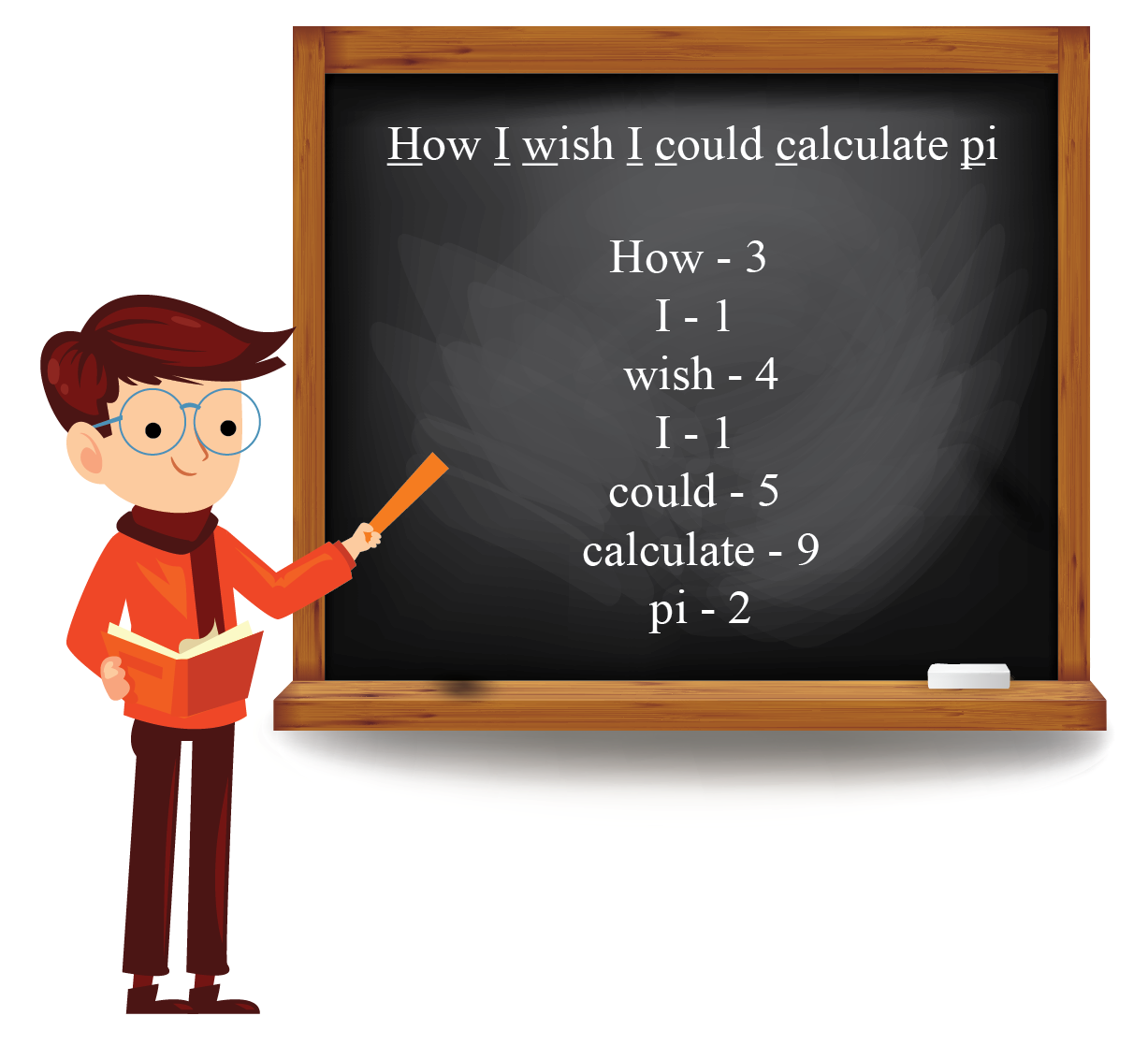pi number approximated to 6 decimal place is

 $$\pi = 3.141592$$

## How to Calculate Pi?

Let us do a small activity to see how to calculate pi.

By the end of this activity, we will know what is pi exactly.

Draw a circle of diameter $$1 \:\text{in}$$.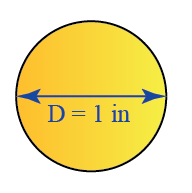Now take a thread and place it along the border of the circle (the circumference).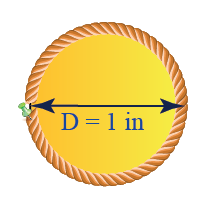Now place the thread on the ruler and note the length.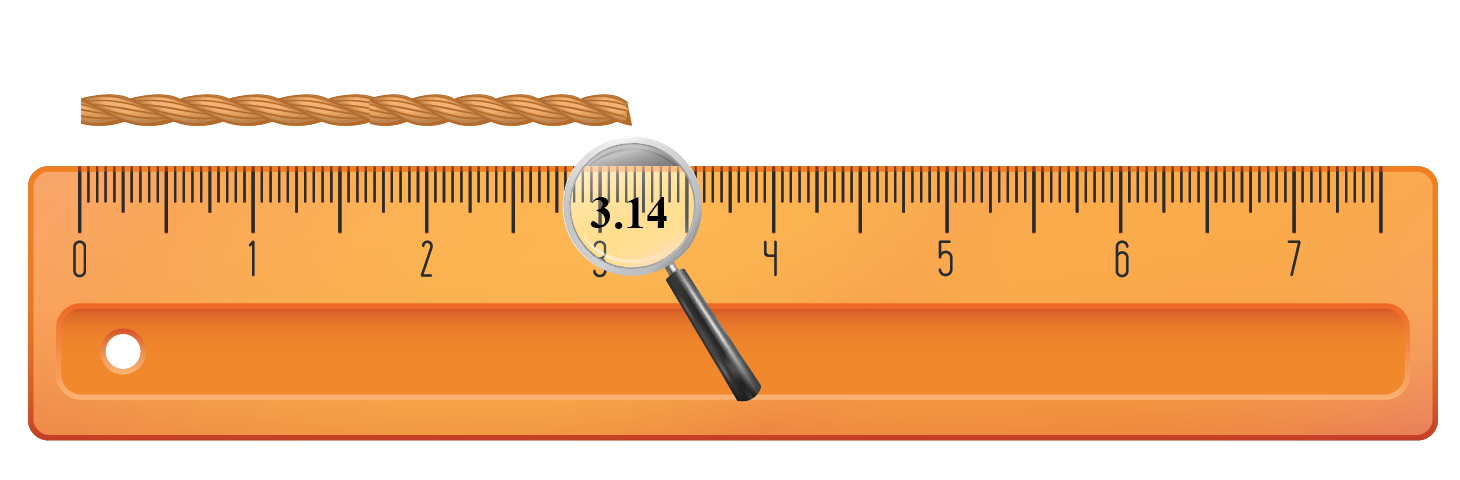Repeat the process with diameters of $$2 \:\text{in}$$, $$3 \:\text{in}$$, $$4 \:\text{in}$$ and  $$5 \:\text{in}$$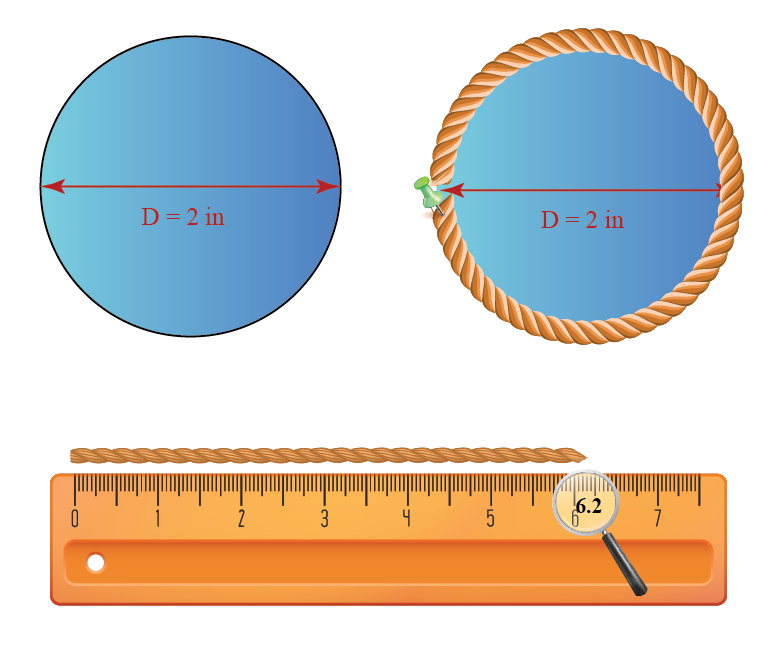Record your observations in the table.

Diameter Circumference $$\dfrac{\textbf{Circumference}}{\textbf{Diameter}}$$
$$1 \: \text{in}$$   $$3.1\: \text{in}$$ $$\frac{3.1}{1} = 3.1$$
$$2\: \text{in}$$   $$6.2\: \text{in}$$ $$\frac{6.2}{2} = 3.1$$
$$3\: \text{in}$$   $$9.3\: \text{in}$$ $$\frac{9.3}{3} = 3.1$$
$$4\: \text{in}$$   $$12.4\: \text{in}$$ $$\frac{12.4}{4} = 3.1$$
$$5\: \text{in}$$   $$15.5\: \text{in}$$ $$\frac{15.5}{5} = 3.1$$

We can observe that the ratio of circumference to diameter is always the same. This answers our question what is pi ($$\pi$$).

## 100 Decimal Places of Pi

### Value of Pi in Decimal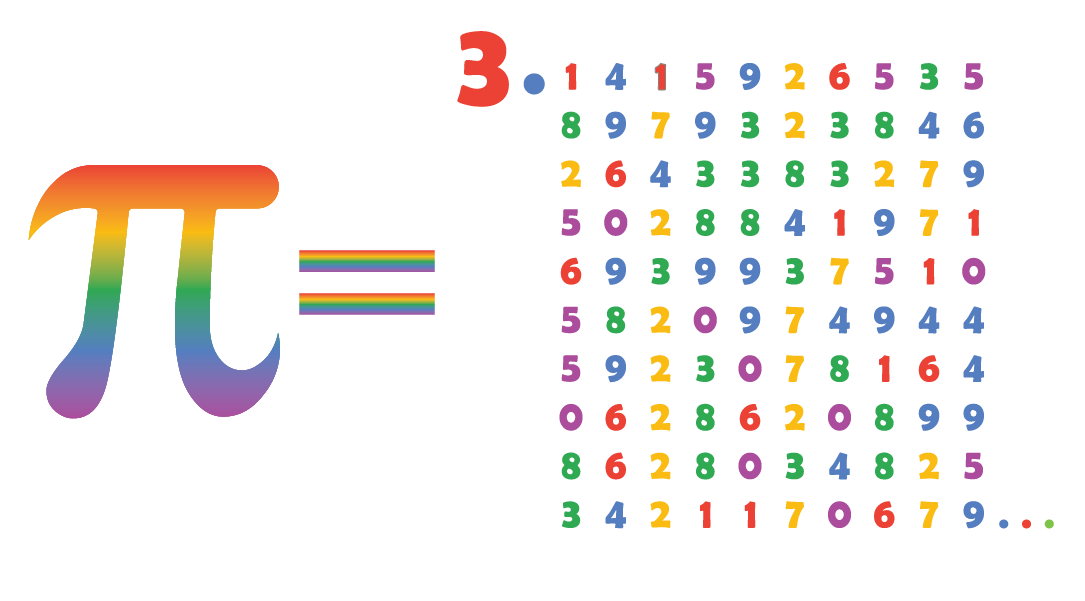The value of pi is an irrational number.

Pi number is non-terminating and non-recurring.

Pi value approximated to $$100$$ decimal places is

$\begin{matrix}3.1415926535 &8979323846 &2643383279 \\ 5028841971 &6939937510 &5820974944 \\ 5923078164 &0628620899 &8628034825 \\ 3421170679 \end{matrix}$

For ease of calculations, it is often approximated to $$3.14$$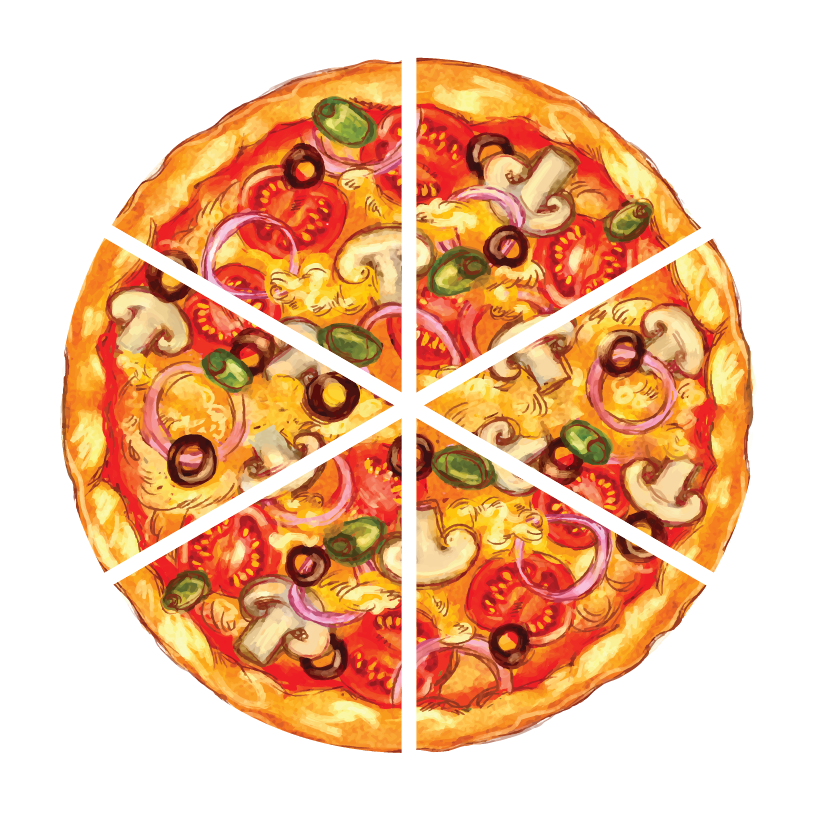Solution:

\begin{align}&= \frac{\text{Diameter}}{2}\\ &= \frac{14}{2} \\ &= 7 \:\text{in} \end{align}

Area of the pizza

\begin{align} &= \pi\: r^2 \\ &= \frac{22}{7} \times 7 \times 7 \\ &= 154\: \text{in}^2 \end{align}

Thus, total area of the pizza is $$154\: \text{cm}^2$$

Area of a slice of the pizza

\begin{align} &= \frac{\text{Area of the pizza}}{\text{Number of slices}}\\ &=\frac{154}{6} \\ &= 25.66 \:\text{in}^2 \end{align}

 $$\therefore \;$$ Area of a slice of pizza $$= 25.66 \:\text{in}^2$$
 Example 2

John had to replace a leaky pipe. He measured $$44 \:\text{mm}$$ around the outside of the pipe. What is the diameter of the pipe he should buy?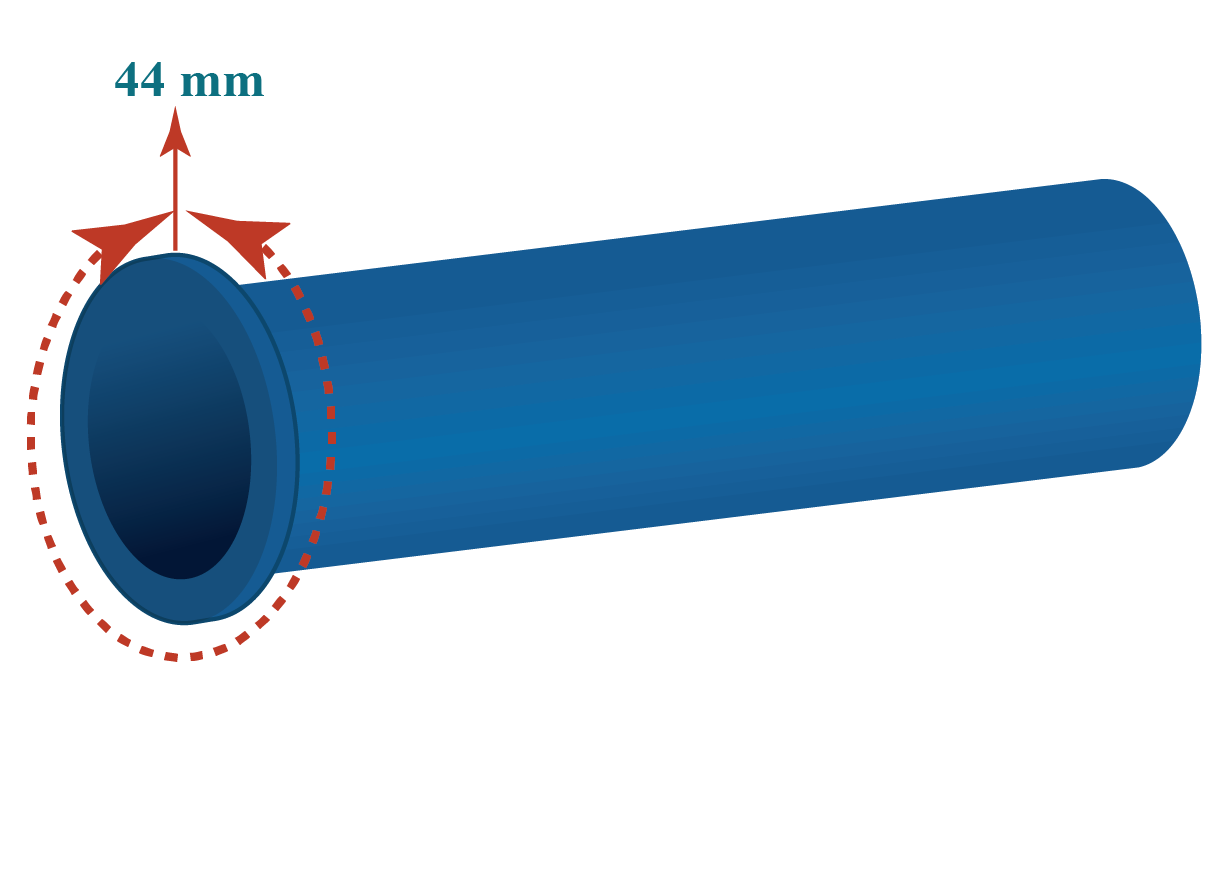Solution:

Circumference of the given pipe is $$44 \:\text{mm}$$

\begin{align} &= 2 \pi\text{r}\\ 44 &= 2 \times\pi \times \text{r} \\ 44 &= 2 \times \frac{22}{7} \times {r} \\ \therefore \text{r} &= 7 \:\text{mm} \end{align}

Therefore, diameter of the pipe

\begin{align} &= 2 \times \text{r}\\ &= 2 \times 7 \\ &= 14 \:\text{mm}\\ \text{D} &= 14 \:\text{mm} \end{align}

 $$\therefore$$ Diameter of the pipe $$= 14 \text{ mm}$$
 Example 3

Betty measured the diameter and circumference of a hula loop accurately.

The diameter was $$113 \:\text{in}$$ and the circumference was $$355 \:\text{in}$$

She used these measurements and calculated $$\pi$$. Is her value close to the real value of $$\pi$$?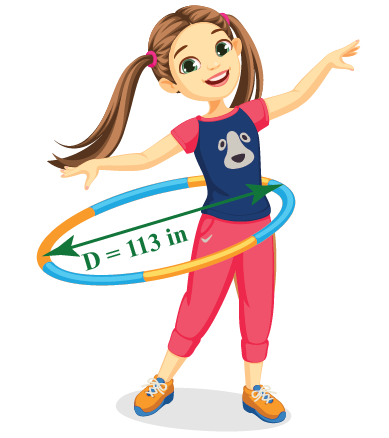Solution:

We know that $$\pi$$

\begin{align} &= \frac{\text{Circumference}}{\text{Diameter}}\\ &= \frac{355}{113} \\ &= 3.1415929 \end{align}

 $$\therefore \;$$\begin{align} \frac{355}{113} \end{align} is very close to the pi value.
 Example 4

Ria goes jogging in a park daily.

The park is shaped semicircular as shown. The diameter of the park is $$70\: \text{yd}$$.

If she goes around the park $$10$$ times. How much distance does she cover?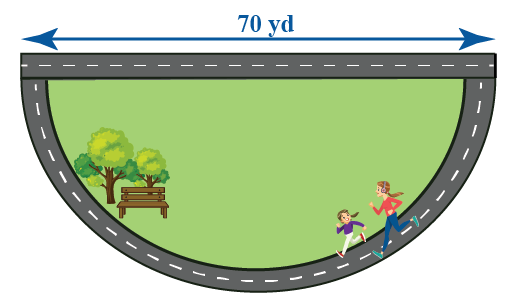Solution:

Perimeter (circumference) of the circle

\begin{align} &= \pi \times \text{d} \\ &= \pi \times 70 \\ &= 70 \pi \end{align}

Perimeter (circumference) of the semi circle

\begin{align} &= \frac{\text{circumference}}{2} + \text{diameter} \\ &= \frac{70 \pi}{2} + 70 \\ &= 35 \pi +70 \\ &= 35 \times \frac{22}{7} + 70 \\ &= 110 +70\\ &= 180 \: \text{yd} \end{align}

Ria goes around the park $$10$$ times.

Therefore, the total distance covered by her

\begin{align} &= 180 \times 10 \\ &= 1800 \text{ yd} \\ \end{align}

 $$\therefore \;$$Ria covers a distance of 1800 yd.Tips and Tricks
1. "How I Wish I Could Calculate Pi" - rembember this line to recall the value of pi (How - 3, I - 1, Wish - 4 , I - 1 Could - 5, Calculate - 9 Pi - 2) $$\pi = 3.141592$$
2. Based on the problem, for ease of calculation, use $$\pi$$ value as $$\frac{22}{7}$$ or $$3.141592$$

## Interactive Questions

Here are a few problems related to pi

## Let's Summarize

We hope you enjoyed learning about What is pi ? with the simulations and practice questions. Now you will be able to easily solve problems on What is pi, pi symbol, pi number, pi circle.

At Cuemath, our team of math experts is dedicated to making learning fun for our favorite readers, the students!

Through an interactive and engaging learning-teaching-learning approach, the teachers explore all angles of a topic.

Be it worksheets, online classes, doubt sessions, or any other form of relation, it’s the logical thinking and smart learning approach that we at Cuemath believe in.

## 1. What are the first 100 digits of Pi?

The first 100 digits of Pi are

 3.14159 8979323846 2643383279 5.02884e+09 6939937510 5820974944 5.92308e+09 0628620899 8628034825 3.42117e+09

## 2. What is Pi exactly?

Pi in mathematics is a constant which is the ratio of the circumference of a circle to its diameter. It is an irrational number often approximated to 3.14159

## 3. Will Pi ever end?

Pi value will never end. It is an irrational number. It is a non-terminating and non-recurring number.

## 4. Why is Pi important?

• Pi in maths is used to calculate the area and circumference of circles.
• Any circular shape is dependent on the pi value.
• $$\pi$$ is found in many formulae in trigonometry to examine the relationship between the lengths and angles of triangles and in geometry where we study about shapes, sizes, relative positions, and the properties of space.
• It is also used extensively in the field of architecture and robotics.
• Pi$$(\pi$$) is considered very important and hence, March 14 is celebrated as Pi day.

More Important Topics
Numbers
Algebra
Geometry
Measurement
Money
Data
Trigonometry
Calculus